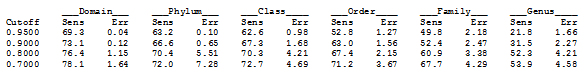Taxonomy confidence measures

The UTAX algorithm generates taxonomy predictions with confidence estimates specified as a value in the range 0.0 to 1.0.

The definition and interpretation of a taxonomy prediction confidence estimate is not as simple as it might appear. Ideally, the error rate of predictions with confidence 0.9 should be approximately 10%, but in practice the error rate depends on the query dataset and on unknown characteristics of the reference dataset. It would be nice to calculate a p-value, but this is tricky because we need two statistical models: one for the hypothesis we are testing plus a null model in which the hypothesis is false and the observation occurs by chance. I don't have a clue how to do this for taxonomy predictions.

Most taxonomy prediction algorithms don't provide a confidence estimate, including GAST, the default QIIME method (assign_taxonomy.py ‑m uclust) and the mothur Classify_seqs command with method=knn. A notable exception is the RDP Naive Bayesian Classifier (RDP) which reports a confidence value obtained by boostrapping. This was an important improvement over previous methods and is a good reason why RDP is currently the most widely-used algorithm for 16S taxonomy prediction. However, everyone agrees that the RDP bootstrap value should not be interpreted as indicating the probabllity that the prediction is correct (which would be 100% minus the estimated error probability). The authors claim that for 16S sequences shorter than 250nt, a bootstrap threshold of 50% gives accurate results to genus level, claiming accuracies from 79% to 100% depending on the V region (see discussion and table under "Confidence threshold" at https://rdp.cme.msu.edu/classifier/class_help.jsp). If this result is valid, the error rate at 50% bootstrap is presumably much less than 50%. However, I believe their "leave-one-out" validation seriously under-estimates error rates on real data (for discussion see validating taxonomy classifiers). On my tests, I find a 33% error rate for genus predictions by RDP on the V3-V5 segment (~530nt) at 50% bootstrap cutoff. At 100% bootstrap confidence, the error rate is 8%.

So, how to define a confidence value that is a reasonable prediction of error rates on real data? The UTAX algorithm does this by a training procedure which splits a reference dataset at different taxonomic levels into query and reference subsets to create a balanced combination of scenarios where taxa each level (genus, family...) are present / not present. This is designed to measure the effects of novel taxa which are missing from the database, which is a serious problem with microbial marker gene reference databases. The confidence value is defined to be the probability that a prediction with a given score is correct, summing over all predictions from all splits.

In practice, predictions with confidence values are typically used to set a threshold. This adds another complication -- we're not just concerned with the error rate exactly at the threshold, we would like to know the total rate for all predictions at or above the chosen value. Even in the ideal situation where 0.9 means 10% error, setting a threshold of 0.9 allows confidence values of 0.9, 0.85, 0.99 etc. If most of the predictions are around 0.90 to 0.92 then the error rate will be worse than if most of them are 0.99 with only a few in the low 0.9s. So, even if confidence=0.9 does give an error rate of ~10% on a given query set, then confidence0.9 should give an rate less than 10%, perhaps much less, and the threshold can be interpreted as a pessimistic lower bound on the cumulative error rate of the predictions that are kept.

To provide guidance on setting a threshold, UTAX reports the sensitivity and error rates obtained during training at a series of cutoffs 0.95, 0.9 etc. To get this report, use the -report option of makeudb_utax (example below).